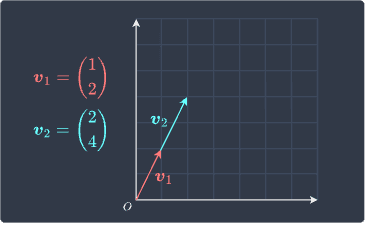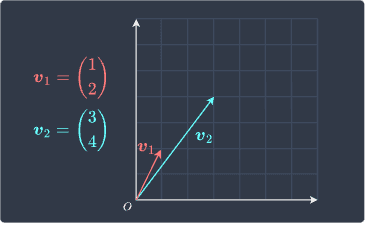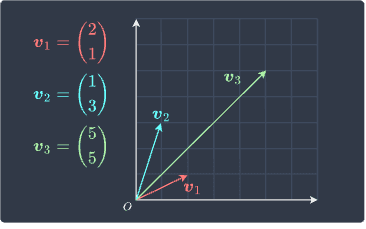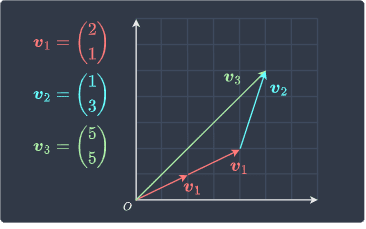search
Search
Unlock 100+ guides
search toc
close
account_circle
Profile
exit_to_app
Sign out
search
keyboard_voice
close
Searching Tips
Search for a recipe:
"Creating a table in MySQL"
Search for an API documentation: "@append"
Search for code: "!dataframe"
Apply a tag filter: "#python"
Useful Shortcuts
/ to open search panel
Esc to close search panel
to navigate between search results
d to clear all current filters
Enter to expand content previewDoc SearchCode Search BetaSORRY NOTHING FOUND!
mic
Start speaking...Voice search is only supported in Safari and Chrome.
Shrink
Navigate to

# Linear Dependence and Independence in Linear Algebra

schedule Aug 10, 2023
Last updated
local_offer
Linear Algebra
Tags
Definition.

# Linear combinations of vectors

If $\boldsymbol{v}_1$, $\boldsymbol{v}_2$, $\cdots$, $\boldsymbol{v}_n$ are vectors, then their linear combination is defined as follows:

$$c_1\boldsymbol{v}_1+ c_2\boldsymbol{v}_2+ \cdots+ c_n\boldsymbol{v}_n$$

Where $c_1$, $c_2$, $\cdots$, $c_n$ are scalar constants.

Example.

## Expressing a vector as a linear combination of other vectors

Consider the following vectors:

$$\boldsymbol{v}_1= \begin{pmatrix} 3\\4 \end{pmatrix},\;\;\;\;\; \boldsymbol{v}_2= \begin{pmatrix} 1\\2 \end{pmatrix},\;\;\;\;\; \boldsymbol{v}_3= \begin{pmatrix}5\\8\end{pmatrix}$$

Express $\boldsymbol{v}_3$ as a linear combination of $\boldsymbol{v}_1$ and $\boldsymbol{v}_2$.

Solution. The linear combination of $\boldsymbol{v}_1$ and $\boldsymbol{v}_2$ that generates $\boldsymbol{v}_3$ is:

$$\boldsymbol{v}_3=\boldsymbol{v}_1+2\boldsymbol{v}_2 \;\;\;\;\;\;\;\;\;\;\Longleftrightarrow\;\;\;\;\;\;\;\;\;\; \begin{pmatrix} 5\\8 \end{pmatrix}= \begin{pmatrix} 3\\4 \end{pmatrix}+ 2\begin{pmatrix} 1\\2 \end{pmatrix}$$

We say that $\boldsymbol{v}_3$ can be expressed as a linear combination of $\boldsymbol{v}_1$ and $\boldsymbol{v}_2$.

# Linear dependence and independence of two vectors

## Linearly dependent vectors

Consider the following two vectors:Can one vector be expressed as a constant multiple of the other? To answer this question, consider the following equation:

$$\begin{equation}\label{eq:m3vR3xzP1TnBBuSrILn} c\begin{pmatrix} 1\\2 \end{pmatrix} = \begin{pmatrix} 2\\4 \end{pmatrix} \end{equation}$$

Clearly, if we let $c=2$, then the equality holds. This means that if we double the shorter vector, we get the longer vector - this should be clear from the diagram as well.

In fact, recall from theoremlink that as long as the two vectors are pointing in the same direction, there will always exist a constant $c$ that satisfies \eqref{eq:m3vR3xzP1TnBBuSrILn} because multiplying a vector by a constant involves stretching/shrinking the vector but preserving the direction.

Whenever we can express two vectors as a multiple of one another, we say that the two vectors are linearly dependent.

## Linearly independent vectors

Consider the following two vectors:We ask ourselves the same question - can we express one vector as a multiple of the other? Again, we are interested in finding a constant that makes the following equality hold:

$$\begin{equation}\label{eq:oDqV0a3tTqzI5YprYaC} c\begin{pmatrix} 1\\2 \end{pmatrix} = \begin{pmatrix} 3\\4 \end{pmatrix} \end{equation}$$

Clearly, there exists no constant value $c$ that satisfies the equality. Setting $c=3$ will make the first elements match, but the second elements will not match. Similarly, setting $c=2$ will only match the second elements.

The fact that $c$ does not exist should make sense from the diagram because $\boldsymbol{v}_1$ and $\boldsymbol{v}_2$ are pointing in different directions. No matter how much we stretch $\boldsymbol{v}_1$, we will never be able to obtain $\boldsymbol{v}_2$.

Whenever we cannot express two vectors as a constant multiple of one another, we say that the two vectors are linearly independent.

## Deriving the formal definition

### Linearly dependent case

Let's now modify equation \eqref{eq:m3vR3xzP1TnBBuSrILn} slightly and include a constant multiple on the right-hand side as well:

$$\begin{equation}\label{eq:BRDlEdUuNi3ZgHYtFz2} c_1\begin{pmatrix} 1\\2 \end{pmatrix} = c_2\begin{pmatrix} 2\\4 \end{pmatrix} \end{equation}$$

Notice that \eqref{eq:BRDlEdUuNi3ZgHYtFz2} is true when $c_1=c_2=0$. However, this is trivial because shrinking any two vectors to a zero vector will always make them identical. If we can find a pair of non-zero constants $c_1$ and $c_2$ that satisfies the equality, then this implies that we can express one vector using a multiple of the other. To understand why this is true, divide both sides by $c_2$ to get:

$$\frac{c_1}{c_2}\begin{pmatrix} 1\\2 \end{pmatrix}= \begin{pmatrix} 2\\4 \end{pmatrix}$$

Since $c_1$ and $c_2$ are just constants, the fraction can also be treated as a constant. In this case, $c_1=2$ and $c_2=1$ will satisfy the equation. Because we managed to express one vector as a multiple of the other, the two vectors must be linearly dependent.

The key takeaway here is that two vectors are linearly dependent only if there exist non-zero constants for the equality \eqref{eq:BRDlEdUuNi3ZgHYtFz2} to hold.

### Linearly independent case

Similarly, let's now modify \eqref{eq:oDqV0a3tTqzI5YprYaC} from earlier using two constants:

$$\begin{equation}\label{eq:VQbMJFwXncwU5HjWo1t} c_1\begin{pmatrix} 1\\2 \end{pmatrix}= c_2\begin{pmatrix} 3\\4 \end{pmatrix} \end{equation}$$

There exists no pair of non-zero constants that satisfy the above equality. In other words, the only solution to \eqref{eq:VQbMJFwXncwU5HjWo1t} is $c_1=c_2=0$, which is the trivial solution. Because neither of the vectors can be expressed as a linear combination of the other, the vectors must be linearly independent.

This means that two vectors are linearly independent only if the constants have to be all zero for the equality \eqref{eq:VQbMJFwXncwU5HjWo1t} to hold.

### Generalization

We have demonstrated that we can determine whether two vectors $\boldsymbol{v}_1$ and $\boldsymbol{v}_2$ are linearly dependent by checking the existence of non-zero constants that satisfy:

$$\begin{equation}\label{eq:Yc8qA0BuXIwROxIAtXs} c_1\boldsymbol{v_1}= c_2\boldsymbol{v_2} \end{equation}$$

Let's move the vector on the right-hand side to the left-hand side to get:

$$\begin{equation}\label{eq:PxgyGjfIqOXoUf1mtmG} c_1\boldsymbol{v_1}- c_2\boldsymbol{v_2}=\boldsymbol{0} \end{equation}$$

We now convert the sign of $c_2$ to positive:

$$\begin{equation}\label{eq:mSkwupqaA4DqVy0fcn9} c_1\boldsymbol{v_1}+ c_2\boldsymbol{v_2}=\boldsymbol{0} \end{equation}$$

We are allowed to alter the sign of the constants because if there exist constant terms $c_1$ and $c_2$ that satisfy \eqref{eq:mSkwupqaA4DqVy0fcn9}, then there will always exist constant terms $c_1$ and $c_2$ satisfying \eqref{eq:PxgyGjfIqOXoUf1mtmG}, and vice versa. For instance, consider the following equation:

$$c_1\begin{pmatrix} 1\\2 \end{pmatrix} - c_2\begin{pmatrix} 2\\4 \end{pmatrix}= \boldsymbol{0}$$

One solution is $c_1=2$ and $c_2=1$. If we swap the sign of $c_2$, then the solution becomes $c_1=2$ and $c_2=-1$. The constant terms have changed but the actual values they take on do not matter - all we care about when determining the linear dependence of two vectors is whether or not there exist constant terms $c_1$ and $c_2$ such that the equality in \eqref{eq:mSkwupqaA4DqVy0fcn9} holds.

NOTE

For our example, the vectors were in $\mathbb{R}^2$, but they can reside in any finite dimension.

# Linear dependence and independence of more than two vectors

When we have more than two vectors, the question changes from "are the two vectors dependent?" to "are the set of vectors dependent?". If a vector can be expressed as a linear combination of the other vectors in the set, then the set is considered to be dependent. Otherwise, the set is independent.

As an example, consider the following set of three vectors:Here, $\boldsymbol{v}_3$ can be expressed as a linear combination of $\boldsymbol{v}_1$ and $\boldsymbol{v}_2$ like so:

$$\begin{equation}\label{eq:znCaorY29bofgU2SYSI} \boldsymbol{v}_3=2\boldsymbol{v}_1+\boldsymbol{v}_2 \end{equation}$$

Visually, this means that:Therefore, our set of vectors is dependent.

WARNING

Every pair of vectors in this set is independent - yet the set of three vectors is dependent.

Now, let's do what we did earlier and generalize the criterion for linear dependence. Recall that the criterion in the case of two vectors is as follows:

$$c_1\boldsymbol{v}_1+c_2\boldsymbol{v}_2=\boldsymbol{0}$$

For the case when we have three vectors, we can guess that the criterion would be:

$$\begin{equation}\label{eq:KPNLoqNjC0SvplKn6mK} c_1\boldsymbol{v}_1+c_2\boldsymbol{v}_2+c_3\boldsymbol{v}_3=\boldsymbol{0} \end{equation}$$

It turns out that this is precisely the criterion to check for linear dependence of three vectors! Let's now understand why. Suppose there exist $c_1$, $c_2$ and $c_3$ that are not all zeros and satisfy \eqref{eq:KPNLoqNjC0SvplKn6mK}. Let's rewrite \eqref{eq:KPNLoqNjC0SvplKn6mK} such that $\boldsymbol{v}_3$ is the subject:

$$\begin{equation}\label{eq:NhBnLsW2XRQzdLZ97cp} \boldsymbol{v}_3=-\frac{c_1}{c_3}\boldsymbol{v}_1 -\frac{c_2}{c_3}\boldsymbol{v}_2\\ \end{equation}$$

Here, we can regard the fraction coefficients as constants. We have managed to express $\boldsymbol{v}_3$ using some linear combinations of vectors $\boldsymbol{v}_1$ and $\boldsymbol{v}_2$, which means that the set of vectors is linearly dependent.

Now, what if the only coefficients that satisfy equation \eqref{eq:KPNLoqNjC0SvplKn6mK} are all zeros? In such cases, the vectors $\boldsymbol{v}_1$, $\boldsymbol{v}_2$ and $\boldsymbol{v}_3$ disappear and we are left with $\boldsymbol{0}=\boldsymbol{0}$. Because we cannot make $\boldsymbol{v}_1$, $\boldsymbol{v}_2$ or $\boldsymbol{v}_3$ the subject, none of the vectors in our set can be constructed using a linear combination of the other vectors in the set.

To generalize further, instead of just three vectors, what if we had $n$ vectors? The criterion to test for linear dependence would be:

$$\begin{equation}\label{eq:lpuN20Wpqn1PEOzGrWE} c_1\boldsymbol{v}_1+c_2\boldsymbol{v}_2+\cdots+c_n\boldsymbol{v}_n =\boldsymbol{0} \end{equation}$$

What we have derived is a criterion to test for linear dependence given multiple vectors. If there exist coefficients that are not all zero satisfying \eqref{eq:lpuN20Wpqn1PEOzGrWE}, then the set of vectors $\boldsymbol{v}_1$, $\boldsymbol{v}_2$, $\cdots$, $\boldsymbol{v}_n$ are linearly dependent. On the other hand, if the only way to get the zero vector is by having all the constant terms be zero, then we have a linearly independent set.

Definition.

# Formal definition of linear dependence and independence

The set of vectors $\boldsymbol{v}_1$, $\boldsymbol{v}_2$, $\cdots$, $\boldsymbol{v}_n$ is said to be linearly dependent if there exist scalars $c_1$, $c_2$, $\cdots$, $c_n$ that are not all zeros satisfying:

$$\begin{equation}\label{eq:LB5A64eXwsweyudsytL} c_1\boldsymbol{v}_1+c_2\boldsymbol{v}_2+\cdots+c_n\boldsymbol{v}_n =\boldsymbol{0} \end{equation}$$

If the only way for the equality to hold is for all $c_1$, $c_2$, $\cdots$, $c_n$ to equal zero, then the set of vectors $\boldsymbol{v}_1$, $\boldsymbol{v}_2$, $\cdots$, $\boldsymbol{v}_n$ is said to be linearly independent.

Note that the left-hand side, which is a sum of scalar-vector products, is called a linear combination of vectors $\boldsymbol{v}_1$, $\boldsymbol{v}_2$, $\cdots$, $\boldsymbol{v}_n$.

Remark. Instead of the above definition, we could have defined linear dependence and independence as follows:

• a set of vectors is linearly dependent if one vector can be constructed as a linear combination of the other vectors.

• a set of vectors is linearly independent if none of the vectors can be constructed as a linear combination of the other vectors.

The reason why the formal definition is preferred is that equation \eqref{eq:LB5A64eXwsweyudsytL} can be expressed in a more practical way using matrices. We shall get back to this laterlink in this guide.

Example.

## Linearly dependent set in R2

Consider the following set of vectors:

$$\boldsymbol{v}_1= \begin{pmatrix} 3\\2 \end{pmatrix},\;\;\;\; \boldsymbol{v}_2= \begin{pmatrix} 6\\4 \end{pmatrix}$$

Show that $\boldsymbol{v}_1$ and $\boldsymbol{v}_2$ are linearly dependent.

Solution. At first glance, $\boldsymbol{v}_2$ is double of $\boldsymbol{v}_1$, so we immediately know that they are linearly dependent. Let's still go through the steps to come to this conclusion mathematically. The formula to test for linear dependence is:

$$\begin{equation}\label{eq:R2jcsXyswMYpOp4zuNL} c_1 \begin{pmatrix}3\\2\end{pmatrix} + c_2\begin{pmatrix}6\\4\end{pmatrix} =\boldsymbol{0} \end{equation}$$

We can reformulate this as a system of linear equations:

$$\begin{cases} 3c_1+6c_2=0\\ 2c_1+4c_2=0 \end{cases}$$

From the first equation, we know that:

$$\begin{equation}\label{eq:T1uZG54EHUKQ84EhWv5} c_1=-2c_2 \end{equation}$$

Substituting this into the second equation gives:

$$0=0$$

This means that there are infinitely many solutions - any pair of $c_1$ and $c_2$ that satisfies the equality \eqref{eq:T1uZG54EHUKQ84EhWv5} is a valid solution. For instance, $c_1=4$ and $c_2=-2$ is one possible solution. Since there exist coefficients $c_1$ and $c_2$ that are not both zeros and satisfy \eqref{eq:R2jcsXyswMYpOp4zuNL}, we conclude that our vectors are linearly dependent.

Because $\boldsymbol{v}_1$ and $\boldsymbol{v}_2$ are linearly dependent, we can express one vector as a scalar multiple of the other. Let's pick the coefficients $c_1=4$ and $c_2=-2$ because they satisfy \eqref{eq:T1uZG54EHUKQ84EhWv5}. Substituting them into \eqref{eq:R2jcsXyswMYpOp4zuNL} gives:

$$(4)\begin{pmatrix}3\\2\end{pmatrix} +(-2)\begin{pmatrix}6\\4\end{pmatrix} =\boldsymbol{0} \;\;\;\;\;\;\;\;\;\Longleftrightarrow\;\;\;\;\;\;\;\;\; 4\boldsymbol{v}_1 -2\boldsymbol{v}_2 =\boldsymbol{0} \;\;\;\;\;\;\;\;\;\Longleftrightarrow\;\;\;\;\;\;\;\;\; \boldsymbol{v}_2= 2\boldsymbol{v}_1$$

Indeed, $\boldsymbol{v}_2$ can be expressed as $2$ times $\boldsymbol{v}_1$.

Example.

## Linearly independent set in R2

Consider the following pair of vectors:

$$\boldsymbol{v}_1= \begin{pmatrix} 3\\2 \end{pmatrix},\;\;\;\; \boldsymbol{v}_2= \begin{pmatrix} 6\\5 \end{pmatrix}$$

Show that $\boldsymbol{v}_1$ and $\boldsymbol{v}_2$ are linearly independent.

Solution. We can easily tell that $\boldsymbol{v}_2$ cannot be expressed as a scalar multiple of $\boldsymbol{v}_1$ and vice versa. Therefore, the two vectors must be linearly independent. Let's still confirm this mathematically using our criterion:

$$\begin{equation}\label{eq:ClVxXzPeL3eG5xOgjBO} c_1 \begin{pmatrix}3\\2\end{pmatrix} + c_2\begin{pmatrix}6\\5\end{pmatrix}= \boldsymbol{0} \end{equation}$$

We can reformulate this as a system of linear equations:

$$\begin{cases} 3c_1+6c_2=0\\ 2c_1+5c_2=0 \end{cases}$$

From the first row, we have that:

$$\begin{equation}\label{eq:vlpR9E6sNDEy66izBb9} c_1=-2c_2 \end{equation}$$

Substituting this into the second row gives:

$$-4c_2+5c_2=0\;\;\;\;\Rightarrow\;\;\;\;c_2=0$$

From \eqref{eq:vlpR9E6sNDEy66izBb9}, we have that $c_1=0$ as well. Since the only way \eqref{eq:ClVxXzPeL3eG5xOgjBO} can be satisfied is by having the coefficients be $0$, we conclude that the vectors are linearly independent.

Example.

## Linearly dependent set in R3

Consider the following three vectors:

$$\boldsymbol{v}_1= \begin{pmatrix} 2\\4\\3 \end{pmatrix},\;\;\;\;\; \boldsymbol{v}_2= \begin{pmatrix} 1\\2\\2 \end{pmatrix},\;\;\;\;\; \boldsymbol{v}_3= \begin{pmatrix} 4\\8\\7 \end{pmatrix}$$

Show that the vectors are linearly dependent.

Solution. Let's solve for $c_1$, $c_2$ and $c_3$ below:

$$\begin{equation}\label{eq:jobxCfha2MUEOEJEA5i} c_1\begin{pmatrix} 2\\4\\3 \end{pmatrix} +c_2\begin{pmatrix} 1\\2\\2 \end{pmatrix}+ c_3\begin{pmatrix} 4\\8\\7 \end{pmatrix} =\boldsymbol{0} \end{equation}$$

Rewriting this as a system of linear equations:

$$\begin{cases} 2c_1+c_2+4c_3=0\\ 4c_1+2c_2+8c_3=0\\ 3c_1+2c_2+7c_3=0\\ \end{cases}$$

Multiplying the top equation by $2$ gives us the middle equation, which means that they are identical. Since we now only have $2$ equations with $3$ unknowns, one constant term is allowed to vary. This means that there are infinitely many solutions of $c_1$, $c_2$ and $c_3$ that satisfy \eqref{eq:jobxCfha2MUEOEJEA5i}. Because there exists a set of non-zero coefficients that satisfies \eqref{eq:jobxCfha2MUEOEJEA5i}, the three vectors must be linearly dependent.

Example.

## Linearly independent set in R3

Consider the following three vectors:

$$\boldsymbol{v}_1= \begin{pmatrix}1\\0\\0\end{pmatrix},\;\;\;\;\; \boldsymbol{v}_2= \begin{pmatrix}0\\1\\0\end{pmatrix},\;\;\;\;\; \boldsymbol{v}_3= \begin{pmatrix}0\\0\\1\end{pmatrix}$$

Show that the set of vectors is linearly independent.

Solution. By inspection, these vectors are linearly independent because we cannot construct any of these vectors using the other vectors. Let's still solve for $c_1$, $c_2$ and $c_3$ below:

$$\begin{equation}\label{eq:yFQuisYj2aZCWXuWXmA} c_1\begin{pmatrix}1\\0\\0\end{pmatrix} +c_2\begin{pmatrix}0\\1\\0\end{pmatrix}+ c_3\begin{pmatrix}0\\0\\1\end{pmatrix} =\boldsymbol{0} \end{equation}$$

This corresponds to the following system of linear equations:

$$\begin{cases} c_1=0\\c_2=0\\c_3=0\\ \end{cases}$$

Because the only coefficients that satisfy \eqref{eq:yFQuisYj2aZCWXuWXmA} are all zeros, our set of vectors must be linearly independent.

Theorem.

# Expressing linear combinations using matrix-vector product

Consider the following linear combinations:

$$x_1\boldsymbol{a}_1+ x_2\boldsymbol{a}_2+ \cdots+ x_n\boldsymbol{a}_n$$

Where $x_i\in\mathbb{R}$ and $\boldsymbol{a}_i\in\mathbb{R}^m$ for $i=1,2,\cdots,n$. This can be expressed as a matrix-vector product:

$$\begin{equation}\label{eq:y0B5oUWXjxeqQX1HUIN} x_1\boldsymbol{a}_1+ x_2\boldsymbol{a}_2+ \cdots+ x_n\boldsymbol{a}_n= \begin{pmatrix} \vert&\vert&\vert&\vert\\ \boldsymbol{a}_1&\boldsymbol{a}_2&\cdots&\boldsymbol{a}_n\\ \vert&\vert&\vert&\vert \end{pmatrix} \begin{pmatrix} x_1\\ x_2\\ \vdots\\ x_n\\ \end{pmatrix}= \boldsymbol{A}\boldsymbol{x} \end{equation}$$

Here, $\boldsymbol{A}$ is a matrix whose columns are composed of vectors $\boldsymbol{a}_i$.

Proof. This theorem is equivalent to theoremlink.

Example.

## Linear combination of three vectors

Consider the following linear combination of vectors:

$$\begin{equation}\label{eq:NN895vYDrnrfWzljlhZ} 2\boldsymbol{a}_1+ 5\boldsymbol{a}_2+ \boldsymbol{a}_3 \end{equation}$$

Where the vectors are defined as:

$$\boldsymbol{a}_1=\begin{pmatrix} 1\\ 3\\ 2 \end{pmatrix},\;\;\;\; \boldsymbol{a}_2=\begin{pmatrix} 9\\ 3\\ 2 \end{pmatrix},\;\;\;\; \boldsymbol{a}_3=\begin{pmatrix} 5\\ 4\\ 2 \end{pmatrix}$$

Express \eqref{eq:NN895vYDrnrfWzljlhZ} as a matrix-vector product.

Solution. We can directly use theoremlink to express equation \eqref{eq:NN895vYDrnrfWzljlhZ} as a matrix-vector product:

\begin{align*} 2\boldsymbol{a}_1+5\boldsymbol{a}_2+\boldsymbol{a}_3 &=\begin{pmatrix} \vert&\vert&\vert\\ \boldsymbol{a}_1&\boldsymbol{a}_2&\boldsymbol{a}_3\\ \vert&\vert&\vert \end{pmatrix} \begin{pmatrix} 2\\5\\1 \end{pmatrix}\\&= \begin{pmatrix} 1&9&5\\3&3&4\\2&2&2 \end{pmatrix} \begin{pmatrix} 2\\ 5\\ 1 \end{pmatrix} \end{align*}
Theorem.

# Formal definition of linear dependence and independence in matrix-vector form

Recall that the criterion of linear dependence is:

$$c_1\boldsymbol{v}_1+c_2\boldsymbol{v}_2+\cdots+c_n\boldsymbol{v}_n =\boldsymbol{0}$$

Using theoremlink, we can rewrite this in terms of a matrix-vector product:

$$\begin{equation}\label{eq:kTGrGeyO2ZMz4lDlHFg} \boldsymbol{0}= \begin{pmatrix} \vert&\vert&\vert&\vert\\ \boldsymbol{v}_1&\boldsymbol{v}_2&\cdots&\boldsymbol{v}_n\\ \vert&\vert&\vert&\vert \end{pmatrix} \begin{pmatrix} c_1\\ c_2\\ \vdots\\ c_n\\ \end{pmatrix} \end{equation}$$

Note the following:

• if the only solution is $c_1=c_2=\cdots=c_n=0$, then the set of vectors $\boldsymbol{v}_1$, $\boldsymbol{v}_2$, $\cdots$, $\boldsymbol{v}_n$ is independent.

• otherwise, the set is linearly dependent.

Remark. The matrix-vector form has two advantages:

• we can easily use a computer program to solve for the coefficients.

• we can use Gaussian elimination to solve for the coefficients. We will see an example of this later.

Example.

# Showing linear independency using Gaussian elimination

Consider the following two vectors:

$$\boldsymbol{v}_1=\begin{pmatrix} 3\\2 \end{pmatrix},\;\;\;\;\; \boldsymbol{v}_2=\begin{pmatrix} 6\\2 \end{pmatrix}$$

Show that these two vectors are linearly independent.

Solution. Using matrix-vector notation, the criterion for linear dependence is:

$$\begin{equation}\label{eq:r436dJsdMikBCUppLTT} \begin{pmatrix} 0\\ 0 \end{pmatrix}= \begin{pmatrix} 3&6\\ 2&2 \end{pmatrix} \begin{pmatrix} c_1\\ c_2 \end{pmatrix} \end{equation}$$

We can ignore the zero vector on the left during Gaussian elimination because performing any elementary row operationlink (e.g. summation, multiplication) will not have an impact on zeros. Therefore, we can focus on row-reducing the matrix term only:

$$\begin{equation}\label{eq:Gw1enp022fFpeSRSviZ} \begin{pmatrix} 3&6\\ 2&2\\ \end{pmatrix} \sim \begin{pmatrix} 1&2\\ 1&1\\ \end{pmatrix} \sim \begin{pmatrix} 1&2\\ 0&1\\ \end{pmatrix} \sim \begin{pmatrix} 1&0\\ 0&1\\ \end{pmatrix} \end{equation}$$

This means that \eqref{eq:r436dJsdMikBCUppLTT} can be reformulated as below since they share the same solution set:

$$\begin{equation}\label{eq:Z4UDMRfkEtliiAfOQ14} \begin{pmatrix} 0\\ 0 \end{pmatrix}= \begin{pmatrix} 1&0\\ 0&1\\ \end{pmatrix} \begin{pmatrix} c_1\\ c_2\\ \end{pmatrix} \end{equation}$$

The only way the above can be true is if $c_1$ and $c_2$ are both equal to zero. This means that the original vectors $\boldsymbol{v}_1$ and $\boldsymbol{v}_2$ are linearly independent.

Example.

# Showing linear dependency using Gaussian elimination

Consider the following vectors:

$$\boldsymbol{v}_1= \begin{pmatrix} 2\\3\\1 \end{pmatrix},\;\;\;\;\; \boldsymbol{v}_2= \begin{pmatrix} 1\\1\\3 \end{pmatrix},\;\;\;\;\; \boldsymbol{v}_3= \begin{pmatrix} 7\\9\\11 \end{pmatrix}$$

Show that this set of vectors is linearly dependent. Also, express $\boldsymbol{v}_3$ as a linear combination of $\boldsymbol{v}_1$ and $\boldsymbol{v}_2$.

Solution. The criterion for linear dependence is:

$$\begin{equation}\label{eq:Yp1nezlKJNYTxna9JZi} c_1\boldsymbol{v}_1 +c_2\boldsymbol{v}_2 +c_3\boldsymbol{v}_3 =\boldsymbol{0} \end{equation}$$

Expressing this in matrix-vector form:

$$\begin{pmatrix} \vert&\vert&\vert\\\boldsymbol{v}_1&\boldsymbol{v}_2&\boldsymbol{v}_3\\\vert&\vert&\vert\\ \end{pmatrix} \begin{pmatrix}c_1\\c_2\\c_3\end{pmatrix}=\boldsymbol{0} \;\;\;\;\;\;\;\;\Longleftrightarrow\;\;\;\;\;\;\;\; \begin{pmatrix}2&1&7\\3&1&9\\1&3&11\end{pmatrix} \begin{pmatrix}c_1\\c_2\\c_3\end{pmatrix}=\boldsymbol{0}$$

Now, we perform row-reduction like so:

$$\begin{pmatrix} 2 & 1 & 7\\ 3 & 1 & 9\\ 1 & 3 & 11 \end{pmatrix} \sim \begin{pmatrix} 2 & 1 & 7\\ 0 & 1 & 3\\ 0 & -5 & -15 \end{pmatrix} \sim \begin{pmatrix} 2 & 1 & 7\\ 0 & 1 & 3\\ 0 & 1 & 3 \end{pmatrix} \sim \begin{pmatrix} 2 & 1 & 7\\ 0 & 1 & 3\\ 0 & 0 & 0 \end{pmatrix} \sim \begin{pmatrix} 2 & 0 & 4\\ 0 & 1 & 3\\ 0 & 0 & 0 \end{pmatrix} \sim \begin{pmatrix} 1 & 0 & 2\\ 0 & 1 & 3\\ 0 & 0 & 0 \end{pmatrix}$$

Because the last row is all zeros, $c_3$ can take on any value. At this point, we already know that this system has infinitely many solutions, which means that the set of vectors is linearly dependent. Therefore, we can express a vector as a linear combination of the other vectors.

For simplicity, let's say $c_3=1$. Using the second row of the reduced row echelon form, we have that $c_2=-3$. Using the first row, we have that $c_1=-2$. Substituting these coefficients into \eqref{eq:Yp1nezlKJNYTxna9JZi} gives us:

$$-2\boldsymbol{v}_1 +-3\boldsymbol{v}_2 +\boldsymbol{v}_3 =\boldsymbol{0}$$

Making $\boldsymbol{v}_3$ the subject:

$$\boldsymbol{v}_3= 2\boldsymbol{v}_1+3\boldsymbol{v}_2$$

We have managed to express $\boldsymbol{v}_3$ as a linear combination of $\boldsymbol{v}_1$ and $\boldsymbol{v}_2$!

thumb_up
thumb_down
Comment
Citation
Ask a question or leave a feedback...
thumb_up
2
thumb_down
0
chat_bubble_outline
0
settings
Enjoy our search
Hit / to insta-search docs and recipes!